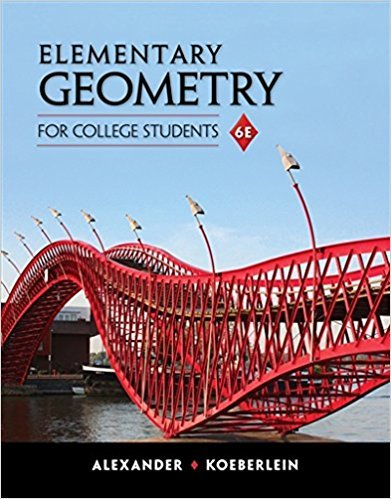×
×

# Solutions for Chapter 1: Line and Angle Relationships## Full solutions for Elementary Geometry for College Students | 6th Edition

ISBN: 9781285195698Solutions for Chapter 1: Line and Angle Relationships

Solutions for Chapter 1
4 5 0 409 Reviews
13
0
##### ISBN: 9781285195698

This textbook survival guide was created for the textbook: Elementary Geometry for College Students, edition: 6. Elementary Geometry for College Students was written by and is associated to the ISBN: 9781285195698. This expansive textbook survival guide covers the following chapters and their solutions. Chapter 1: Line and Angle Relationships includes 57 full step-by-step solutions. Since 57 problems in chapter 1: Line and Angle Relationships have been answered, more than 4074 students have viewed full step-by-step solutions from this chapter.

Key Math Terms and definitions covered in this textbook
• Diagonalizable matrix A.

Must have n independent eigenvectors (in the columns of S; automatic with n different eigenvalues). Then S-I AS = A = eigenvalue matrix.

• Fibonacci numbers

0,1,1,2,3,5, ... satisfy Fn = Fn-l + Fn- 2 = (A7 -A~)I()q -A2). Growth rate Al = (1 + .J5) 12 is the largest eigenvalue of the Fibonacci matrix [ } A].

• Fourier matrix F.

Entries Fjk = e21Cijk/n give orthogonal columns FT F = nI. Then y = Fe is the (inverse) Discrete Fourier Transform Y j = L cke21Cijk/n.

• Hessenberg matrix H.

Triangular matrix with one extra nonzero adjacent diagonal.

• Hilbert matrix hilb(n).

Entries HU = 1/(i + j -1) = Jd X i- 1 xj-1dx. Positive definite but extremely small Amin and large condition number: H is ill-conditioned.

• Identity matrix I (or In).

Diagonal entries = 1, off-diagonal entries = 0.

• Incidence matrix of a directed graph.

The m by n edge-node incidence matrix has a row for each edge (node i to node j), with entries -1 and 1 in columns i and j .

• Left inverse A+.

If A has full column rank n, then A+ = (AT A)-I AT has A+ A = In.

• Left nullspace N (AT).

Nullspace of AT = "left nullspace" of A because y T A = OT.

• Markov matrix M.

All mij > 0 and each column sum is 1. Largest eigenvalue A = 1. If mij > 0, the columns of Mk approach the steady state eigenvector M s = s > O.

• Nilpotent matrix N.

Some power of N is the zero matrix, N k = o. The only eigenvalue is A = 0 (repeated n times). Examples: triangular matrices with zero diagonal.

• Norm

IIA II. The ".e 2 norm" of A is the maximum ratio II Ax II/l1x II = O"max· Then II Ax II < IIAllllxll and IIABII < IIAIIIIBII and IIA + BII < IIAII + IIBII. Frobenius norm IIAII} = L La~. The.e 1 and.e oo norms are largest column and row sums of laij I.

• Nullspace N (A)

= All solutions to Ax = O. Dimension n - r = (# columns) - rank.

• Orthonormal vectors q 1 , ... , q n·

Dot products are q T q j = 0 if i =1= j and q T q i = 1. The matrix Q with these orthonormal columns has Q T Q = I. If m = n then Q T = Q -1 and q 1 ' ... , q n is an orthonormal basis for Rn : every v = L (v T q j )q j •

• Outer product uv T

= column times row = rank one matrix.

• Partial pivoting.

In each column, choose the largest available pivot to control roundoff; all multipliers have leij I < 1. See condition number.

• Random matrix rand(n) or randn(n).

MATLAB creates a matrix with random entries, uniformly distributed on [0 1] for rand and standard normal distribution for randn.

• Schur complement S, D - C A -} B.

Appears in block elimination on [~ g ].

• Spanning set.

Combinations of VI, ... ,Vm fill the space. The columns of A span C (A)!

• Trace of A

= sum of diagonal entries = sum of eigenvalues of A. Tr AB = Tr BA.

×